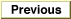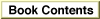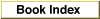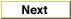# Legacy DocumentImportant: The information in this document is obsolete and should not be used for new development.

Inside Macintosh: QuickDraw GX Environment and Utilities /
Chapter 8 - QuickDraw GX Mathematics / QuickDraw GX Mathematics Reference
Mathematical Functions / Fixed-Point Operations

### FractCubeRoot

You can use the `FractCubeRoot` function to calculate the cube root of a `fract` number.

```fract FractCubeRoot (fract source);
```
`source`
The `fract` number for which the cube root is required.
function result
The cube root of the `fract number. This number is a signed value.`
##### DESCRIPTION
The `FractCubeRoot` function returns the cube root of a `fract` number.